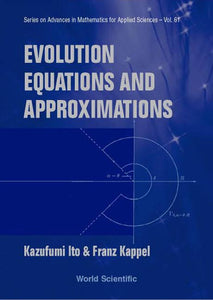# Evolution Equations And Approximations

Kazufumi Ito

Format: Print Book

ISBN: 9789812380265

• SGD 225.77
Unit price per
Tax included.

This book presents an approximation theory for a general class of nonlinear evolution equations in Banach spaces and the semigroup theory, including the linear (Hille-Yosida), nonlinear (Crandall-Liggett) and time-dependent (Crandall-Pazy) theorems.The implicit finite difference method of Euler is shown to generate a sequence convergent to the unique integral solution of evolution equations of the maximal monotone type. Moreover, the Chernoff theory provides a sufficient condition for consistent and stable time integration of time-dependent nonlinear equations. The Trotter-Kato theorem and the Lie-Trotter type product formula give a mathematical framework for the convergence analysis of numerical approximations of solutions to a general class of partial differential equations. This book contains examples demonstrating the applicability of the generation as well as the approximation theory.In addition, the Kobayashi-Oharu approach of locally quasi-dissipative operators is discussed for homogeneous as well as nonhomogeneous equations. Applications to the delay differential equations, Navier-Stokes equation and scalar conservation equation are given.

Format: Hardcover
No of Pages: 520
Imprint: World Scientific
Publication date: 20020528
Series: Series On Advances In Mathematics For Applied Sciences